## Four or Five Band Resistor color code calculator

Code type: 4 band code    5 band code
Resistance:     Select Tolerance:
The colour code is: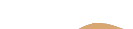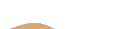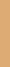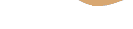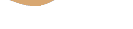Select Value Series: E12    E24    E48    E96    E192
The nearest preferred resistor value is:  Ohms

This calculator solves for 4, 5 band resistors and is quite simple to use. First：Select a Band Resistor, Second click and select color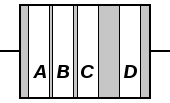To distinguish left from right there is a gap between the C and D bands.

• band A is first significant figure of component value (left side)
• band B is the second significant figure (Some precision resistors have a third significant figure, and thus five bands.)
• band C is the decimal multiplier
• band D if present, indicates tolerance of value in percent (no band means 20%)

For example, a resistor with bands of yellow, violet, red, and gold will have first digit 4 (yellow in table below), second digit 7 (violet), followed by 2 (red) zeros: 4,700 ohms. Gold signifies that the tolerance is ±5%, so the real resistance could lie anywhere between 4,465 and 4,935 ohms.

Thinkcalculator.com provides you helpful and handy calculator resources.